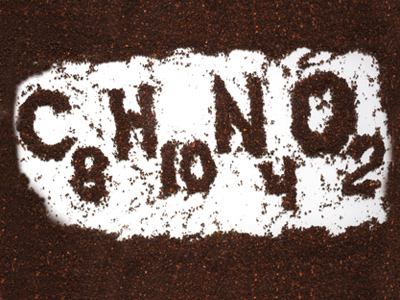C8H10N4O2 is the chemical formula for caffeine.

# Balancing Symbol Equations (H)

Chemistry is all about chemical reactions. Scientists find equations, using either words or symbols, a handy tool to help them understand exactly what is going on in chemical reactions. Balancing these equations is a vital skill for any chemist and necessary for a high grade in GCSE Chemistry. This quiz will test students’ understanding and knowledge when faced with balancing symbol equations.

In chemistry, equations show what is happening in a chemical reaction. The starting materials are known as the reactants (sometimes called reagents) and the new materials are called the products. Scientists use balanced symbol equations in order to describe chemical reactions. Calculations of reacting quantities can be made using these balanced equations so they are of great importance to anyone involved with chemistry. At first, balancing symbol equations can seem daunting but if you follow a few simple rules, it becomes a lot easier!

At GCSE, it is best to begin with a word equation so that you can quickly see that you have all of the reactants and products. Underneath that, write down the correct formula for each of the chemicals. This is the crucial step - if any of the formulae are wrong, the final equation may never balance. Once that is done, you can count the atoms of the individual elements on both sides of the reaction. Make two lists on a piece of rough paper, one for the atoms on the reagents side and another for the products. The Law of Conservation of Matter means that the products must contain exactly the same number and type of atoms as the reactants, but not joined together in the same way. When the two lists contain exactly the same numbers and types of atoms, the equation is balanced.

You can then start to balance the equation. Some equations will automatically be balanced but the majority won't be. You cannot change the formulae (unless you realise one of them is wrong), all you can do is to change the quantities of each formula. You do this by placing a number in front of the formulae as required e.g. 2NaCl or 3H2O. This doubles, trebles, quadruples etc. ALL of the elements in the formula. NaCl means that you have one atom of sodium and one of chlorine. Placing a 2 in front doubles the numbers of both the sodium and chlorine atoms. This works with every formula. As you add a number, you need to update your lists of atoms.

Balancing symbol equations, which is only required on the higher tier paper, is much harder to describe than it is to do! Practice makes perfect so try your hand at this quiz and sharpen your equation balancing skills!

1.
Choose the correctly balanced chemical equation from the list.
CH4 + 2O2 → CO2 + H2O
CH4 + 3O2 → CO2 + 2 H2O
CH4 + 2O2 → CO2 + 2 H2O
CH4 + 2O2 → CO2 + 3 H2O
The complete combustion of methane
2.
Choose the correctly balanced chemical equation from the list.
Fe2O3 + 3CO → 2Fe + 3CO2
2Fe2O3 + 2CO → Fe + 3CO2
Fe2O3 + 3CO → Fe + 2CO2
Fe2O3+ 3CO → Fe + CO2
Two Fe, three C and six O atoms on each side
3.
Choose the correctly balanced chemical equation from the list.
2H2 SO4 + Pb(OH)4 → Pb(SO4)2 + 4H2O
H2 SO4 + Pb(OH)4 → Pb(SO4)2 + 4H2O
2H2 SO4 + Pb(OH)4 → PbSO4 + 4H2O
2H2 SO4 + Pb(OH)4 → Pb(SO4)2 + H2O
Remember, brackets in chemistry work the same as in maths
4.
Choose the correctly balanced chemical equation from the list.
Al + 6HCl → 2AlCl3 + 3H2
2Al + 6HCl → 2AlCl3 + 3H2
Al + HCl → AlCl3 + H2
2Al + HCl → AlCl3 + 3H2
Two Al, six H and six Cl on each side
5.
Choose the correctly balanced chemical equation from the list.
3Na3PO4 + 3HCl → 3NaCl + H3PO4
Na3PO4 + 2HCl → 3NaCl + H3PO4
Na3PO4 + HCl → NaCl + H3PO4
Na3PO4 + 3HCl → 3NaCl + H3PO4
This one looks worse than it is - all that happens is that the Na and the H swap places during the reaction
6.
Choose the correctly balanced chemical equation from the list.
Mg(OH)2 + 2HCl → MgCl2 + H2O
Mg(OH)2 + 2HCl → MgCl2 + 2H2O
Mg(OH)2 + HCl → MgCl2 + H2O
MgOH + 2HCl → MgCl2 + 2H2O
Balancing equations gets easier the more you do
7.
Choose the correctly balanced chemical equation from the list.
TiCl4 + H2O → TiO + 4HCl
TiCl4 + 3H2 → TiO2 + 5HCl
TiCl4 + H2 → TiO2 + HCl
TiCl4 +2H2O → TiO2 + 4HCl
Titanium oxide is used in sun creams
8.
Choose the correctly balanced chemical equation from the list.
2NaBr + Cl2 → 2NaCl + Br2
NaBr + Cl2 → NaCl + Br2
2NaBr + Cl2 → NaCl + Br2
3NaBr + Cl2 → 3NaCl + Br2
One of the halogen displacement reactions
9.
Choose the balanced chemical equation from the list.
C2H6 + 31/2O2 → 2CO2 + 3H2O
C2H6 + 7O2 → 4CO2 + 5H2O
C2H6 + 3O2 → 2CO2 + 3H2O
C2H6 + 31/2O2 → CO2 + 3H2O
It is more common and scientifically correct to use integers (whole numbers) for balancing equations, but you will sometimes see or equations that have been balanced in this way using 1/2. Examples of these are intermediate steps towards writing the final balanced equation, questions testing your knowledge of the Law of Conservation of Matter or occasionally to make calculations of reacting masses slightly easier
10.
Choose the correctly balanced chemical equation from the list.
PCl5 + H2O → H3PO4 + HCl
PCl5 + 4H2O → H3PO4 + HCl
PCl5 + 4H2O → H3PO4 + 5HCl
PCl5 + 4H2O → H3PO4 + 5HC
The reaction of phosphorous pentachloride with water is very violent!